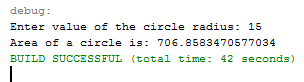# Calculate Area Circle Using Java Example

All programming language is designed to perform a mathematical operation. Java language is bundled with Math library for calculation in a simple and complex problem.

This tutorial will teach you on how your Java program Calculate the Area of a Circle based on user input value. This tutorial will use a Scanner function and an Integer and Double data type for variables. Please follow all the steps to complete this tutorial.

### Calculate the Area of a Circle in Java Steps

1. Create a new class and name it what you want.

2. Above your Main Class, Insert an import code to access the  Scanner library.

[java]import java.util.Scanner;[/java]

3. Declare a scanner and two variables inside your main method.

[java]Scanner input = new Scanner(System.in);
double area;[/java]

4. After step 3, insert the code below for user input.

[java]System.out.print(“Enter value of the circle radius: “);

5. After step 4, insert the code below for the algorithm and output.

System.out.println(“Area of a circle is: ” + area);[/java]

6. Run your program and the output should look like the image below.7. Complete Source Code.

[java]import java.util.Scanner;
public class CalculateCircleArea {
public static void main(String args[]){
Scanner input = new Scanner(System.in);